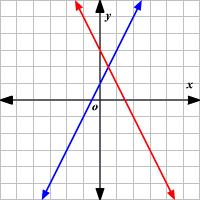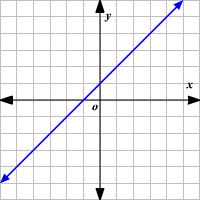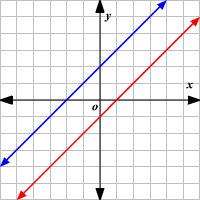# Consistent and Dependent Systems

The two equations $y=2x+5$ and $y=4x+3$ , form a system of equations . The ordered pair that is the solution of both equations is the solution of the system.

A system of two linear equations can have one solution, an infinite number of solutions, or no solution. Systems of equations can be classified by the number of solutions.

If a system has at least one solution, it is said to be consistent .

If a consistent system has exactly one solution, it is independent .If a consistent system has an infinite number of solutions, it is dependent . When you graph the equations, both equations represent the same line.If a system has no solution, it is said to be inconsistent . The graphs of the lines do not intersect, so the graphs are parallel and there is no solution.